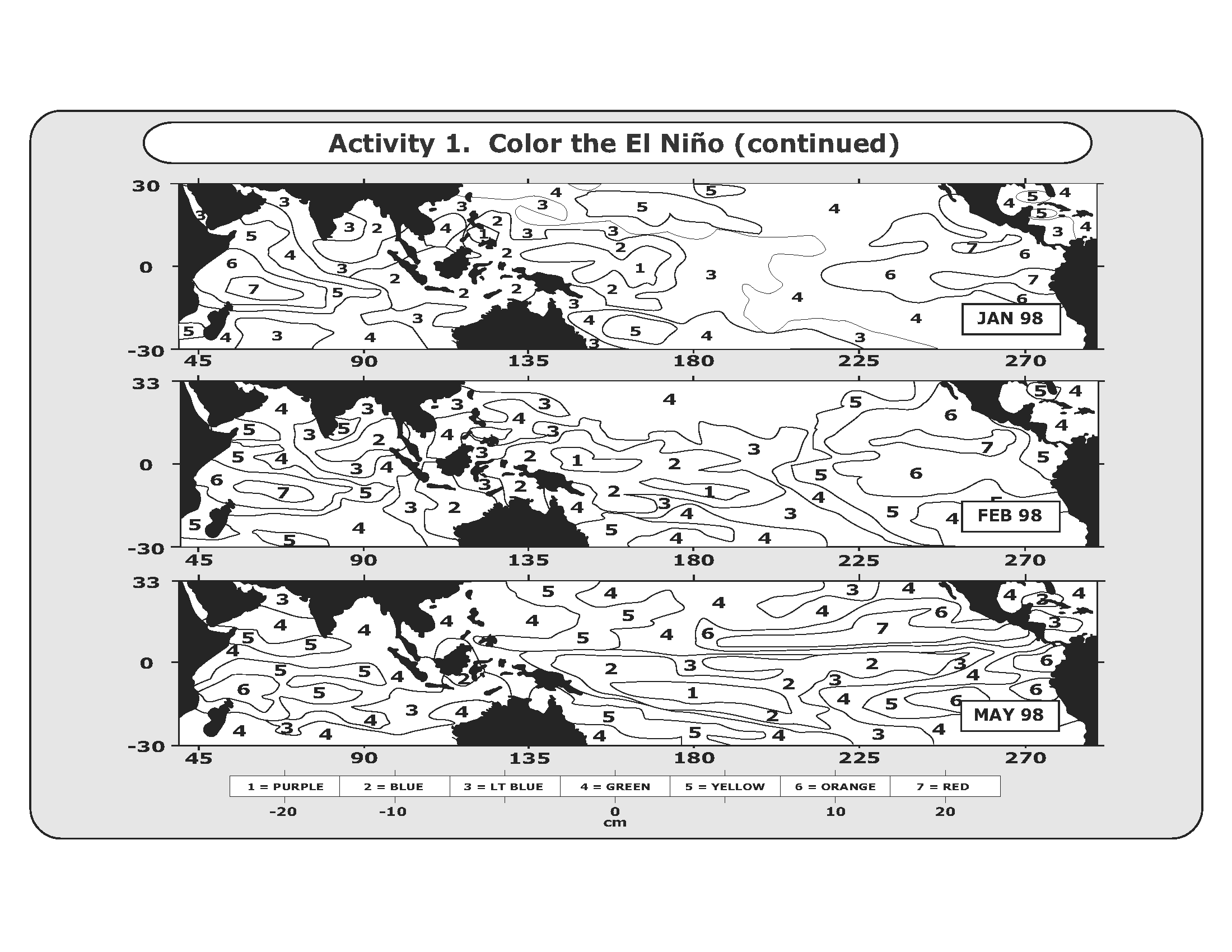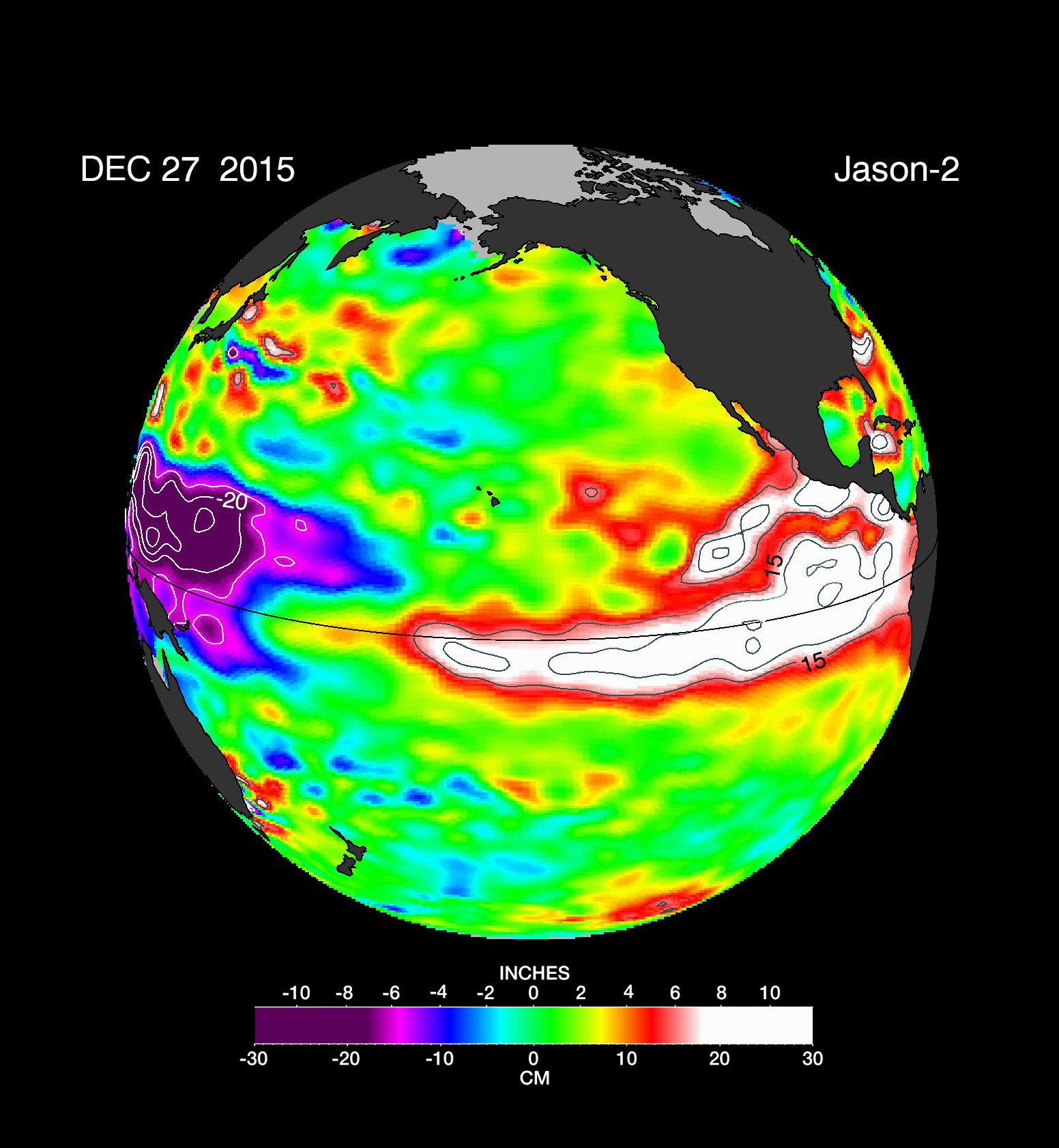The El Niño Poster Model

Students will use coloring sheets to create a color coded model of El Niño. If the Data Literacy Map Cube is used with this, students should color their models first.

Mini Lesson

These pages for this model were taken from The El Niño Poster

Materials Needed:1. Coloring Pages (model pages)
2. Crayons in the following colors:
• Purple
• Blue
• Light Blue
• Green
• Yellow
• Orange
• Red

Have students fill in the color coded coloring sheets or models before answering question set 1.

Question Set 1

1. Each color represents the difference between the normal sea level in centimeters. What is the approximate range of the difference between normal sea level for each color?
2. What do negative numbers represent?
3. What does zero represent?
4. Compare the images from the different months.
5. What differences do you see between March 1997 and May 1997?
6. What differences do you see between May 1997 and November 1997?
7. What differences do you see between November 1997 and January 1998?
8. What differences do you see between January 1998 and February 1998?
9. What differences do you see between February 1998 and May 1998?
10. Explain what happened over the year.

Now examine the image from 2015 and answer question set 2.Question Set 2

Compare the scales for the model pages you colored and the image.

1. What additional color is there in the scale for the image?
2. What height does the additional color represent?
3. Are the ranges for the colors any different? Explain.

Compare the model you made to the image provided.

1. What are the similarities between these two El Niño events?
2. What are the differences between these two El Niño events?